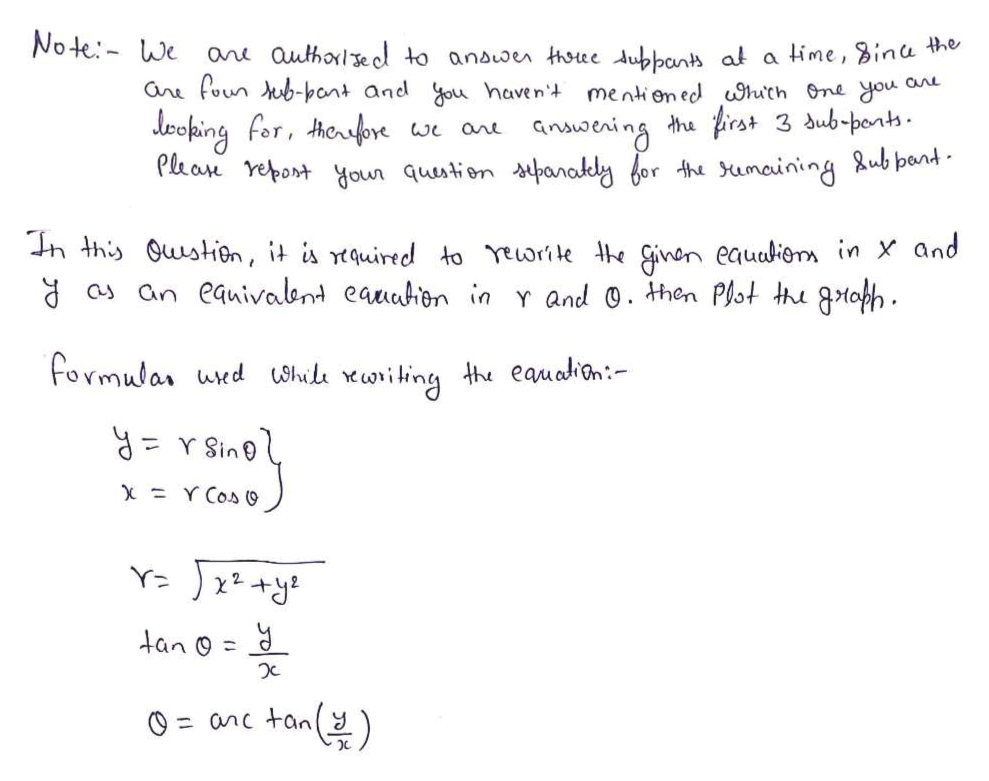# Rewrite the following equations in r and θ as an equivalent equation in x and y; then plot the graph. a) r = 2 sec(θ)b) r = 4c) θ =  π/3d) r = 2 sin(θ)

Question
1 views

Rewrite the following equations in r and θ as an equivalent equation in x and y; then plot the graph.

a) r = 2 sec(θ)

b) r = 4

c) θ =  π/3

d) r = 2 sin(θ)

check_circle

star
star
star
star
star
1 Rating
Step 1help_outlineImage TranscriptioncloseNote We ane authole el to anauoen thoee duppanh at a time, Bina the ane foun ub-pant and ou haven CnL mentioned huth One dou 3 dub-pont Jeoping for, ouore Phan Yepont youn quustion thanakly bor th umcining Aub pant the naening we ane h this Qustion, it is rtquired to Yeorie he inon eauaion in and o an eauivabn eauahen in r and . then Pot thu gtofh Formular ued oil oiting th eauation: X Y Cos (9 tan o (4) anc tan fullscreen
Step 2
Step 3

### Want to see the full answer?

See Solution

#### Want to see this answer and more?

Solutions are written by subject experts who are available 24/7. Questions are typically answered within 1 hour.*

See Solution
*Response times may vary by subject and question.
Tagged in

### Other